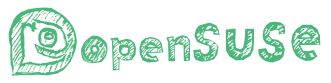# 关于用c语言打印图形的一些问题

``````#!/usr/bin/env ruby

r = 10 # 半径

# 区间, x = -12, 12], y = -12, 12]
12.downto(-12).each do |y|
(-12..12).each do |x|
if r**2 >= x**2 + y**2
print "*"
else
print " "
end
end
puts ""
end

# 区间, x = [0, 12], y = [0, 12]
12.downto(0).each do |y|
(0..12).each do |x|
if r**2 >= x**2 + y**2
print "*"
else
print " "
end
end
puts ""
end``````

``````#include <stdio.h>
#include <stdlib.h>

void print(int row);
void print_formula(int row);
int f(double x, double y, double row);

int main(int argc, char **argv)
{
int row;

scanf("%d", &row);
print(row);
//	print_formula(row);

return EXIT_SUCCESS;
}

void print(int row) {
for(int i = 0; i < row; i++) {
for(int j = 2*i;j < 2*row - 1; j++) {
putchar('*');
}
putchar('\n');
}
}
``````

``````*******
*****
***
*
``````

``````int f(double x, double y, double row) {
return y < row - x/2
}

void print_formula(int row) {
for(int y = 0; y > -row; y--) {
for(int x = 0; x < 2*row - 1 ; x++) {
putchar(f(x, y, row) ? '*' : ' ');
}
putchar('\n');
}
}
``````

``````*
***
*****
*******
``````

``````#!/usr/bin/env ruby
# -*- coding: utf-8 -*-

# x = [0, 6], y = [0, 3]
(0..3).each do |y|
(0..6).each do |x|
if x + 2*y - 6 <= 0
print "*"
else
print " "
end
end
puts ""
end``````